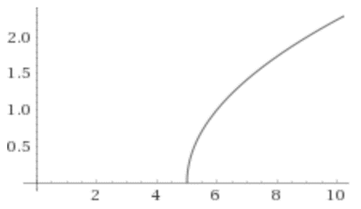# The domain and range of the function and sketch the graph.### Single Variable Calculus: Concepts...

4th Edition
James Stewart
Publisher: Cengage Learning
ISBN: 9781337687805### Single Variable Calculus: Concepts...

4th Edition
James Stewart
Publisher: Cengage Learning
ISBN: 9781337687805

#### Solutions

Chapter 1.1, Problem 39E
To determine

## To find: The domain and range of the function and sketch the graph.

Expert Solution

### Explanation of Solution

Given information:

The given function is,

g(x)=x5

Consider the given equation,

g(x)=x5

The above equation is valid only when,

x50x5

Thus, the domain of the given function is all real numbers x5 .

Consider the graph of the given function,Since the graph is passing above x axis. So, the range of the function is all non-negative real numbers.

### Have a homework question?

Subscribe to bartleby learn! Ask subject matter experts 30 homework questions each month. Plus, you’ll have access to millions of step-by-step textbook answers!